## The g4egq rae postal course - lesson i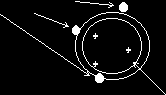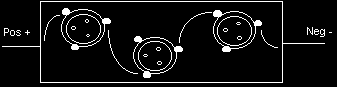LESSON 1 INTRODUCTIONThis lesson introduces you to electricity and the famous law of Mr Ohm. His law is behind many of the little formula that you will encounter in the RAE. If math’s is not your favourite subject read this lesson three times instead of just twice! It is always worth working through my examples to make sure you get the same answer as me. In this lesson, definitions that do not need to be remembered word perfect for the actual RAE, appear between double brackets. . Theory of ElectricityAll substances comprise of molecules and each molecule is made up of one or more atoms. There are about 102 different types of atoms. Everything in the world is made from these atoms or combinations of them. For example, a molecule of water comprises two atoms of hydrogen and one atom of oxygen. (Hence the chemical symbol for water is H20) Each atom comprises a nucleus (in the centre) and one or more orbiting electrons. The electrons each have one negative charge. The atom, as a whole, is normally electrically neutral - the number of positive charges (protons) in the nucleus will equal the number of orbiting electrons. There may also be some neutrons in the nucleus, but as their name suggests they have no charge and only add weight to the atom. The Law of the Charges: Like charges repel and unlike charges attract If, for any reason, an electron is "lost" then its parent atom will become positively charged, as the proton charge will have remained the same. I.E. There will now be more positive charges than negative charges. Due to the above law, the escaping electron (neg. charge) is normally attracted to the parent atom very quickly thus restoring the neutral condition. Often, however, the free electron is attracted to another atom that has lost an electron rather than going back to where it came from. This is not a problem so far as each atom has the correct number of electrons most of the time. In fact, the electrons will jump around randomly from one atom to another. (This mobility of electrons increases if the material is heated. I expect that the little chaps get rather agitated!) But what is electricity If, however, a voltage is connected across the material then the movement of electrons changes from random to an organised drift in one direction. The electrons, being negatively charged, are attracted to the positive terminal of the supply voltage. Electrons jump from one atom to the next making their way across the material. This electron flow is electricity. Insulators and ConductorsIn some materials the electrons are tightly bound to their nucleus and, even when a voltage is applied, will not move. Such materials are called insulators. Materials in which the electrons are loosely bound are called conductors There is no clear division between conductors and Insulators. It depends how tightly or loosely the electrons are bound to their nucleus. Put in other words - it depends on the electron mobility. All materials fall somewhere in the following range:- Good conductors, poor conductors, semiconductors, poor insulators or good insulators. The following examples are given in the same order; I.E. From good conductors to good insulators:- Silver, pure gold, pure copper, aluminium, Iron, soft steel, German silver, mercury, diodes, BC transistors, slate, dry paper, shellac, vulcanised rubber, mica, ebonite, paraffin wax, high grade ceramic, plastic and dry air. It is often convenient to use liquid insulators. For example, mineral oils are often used for insulation in very high power switches. It will prevent arcing by excluding all air. It also conducts away any heat but does not conduct electricity. Which way is the electricity flowing? As we have seen, electricity is the flow of electrons when a voltage is applied to the ends of a conductor. Electrons, being negative, will be attracted to the positive end. Therefore electron current flow will be from Neg. to Pos. Electricity has only been explained fairly recently. Prior to the electron theory it was assumed that electrical current flowed from P0S to NEG. To save re-writing all the books we say that Conventional Current Flow is from Pos. to Neg. Whenever you read the word "current" please remember that it is really short for "conventional current". This does sound rather confusing but In fact it does not cause a problem. Electrical UnitsIt is necessary to be able to measure electricity so various units must be used, The amount of electricity is proportional to the number of electrons actually in motion. Therefore the electron could be used as the unit but the numbers would be very large as each electron charge is so very small. UNIT of QUANTITY OF ELECTRICITY is the COULOMB (Symbol "q") One Coulomb is 6 x 10 18 (Six followed by 18 zeroes!) UNIT of CURRENT FLOW is the AMPERE (Symbol "I”) One Ampere flows when one Coulomb of electricity passes in One Second. I Amps x S Seconds = q Coulomb I.E.: I x S = q or I = q/s UNIT of ELECTRICAL PRESSURE is the VOLT [Symbol V or E (see later)] The Volt is defined as the difference in potential between two points of a conducting wire carrying a constant current of one Amp, when the power dissipated between those two points is one Watt. Where does electricity come from ? In order to maintain a flow of electricity some means of supplying a continuous source of electrons is necessary. E.G. The chemical reaction in a cell or battery of cells. There are two types of cell: primary and secondary. A primary cell produces an electrical voltage by means of a non-reversible chemical reaction. A secondary cell uses a reversible chemical reaction. This means that the secondary cell can be re-charged when it is run down. When it is being recharged electrical energy is converted back into chemical energy. Electricity can also be produced by converting mechanical energy into electrical energy by means of a dynamo (producing direct current) or alternator (producing alternating current). It is worth noting that the word "generator" embraces both the dynamo and the alternator.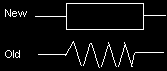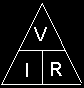The electrical pressure (Voltage) from any of the above forms of supply is called the E.M.F. (Electro-motive force). However, when two points in an electrical circuit do not have the same voltage they are said to have p.d. (potential difference). The unit for both E.M.F. and p.d. is the VOLT. However, the symbol for "Volts E.M.F." is "E" and the symbol for "Volts p.d." is “V". ResistanceResistance is the opposition to the flow of electricity and it is measured in OHMS. The Ohm is defined as that resistance when, with a current of I amp flowing, there is pd. of I Volt across the resistance. The symbol for Ohm is the Greek letter Omega. (Ω) In a circuit diagram a resistor is shown as small rectangle. Until quite recently a zigzag line was used to indicate a resistor.
((If a steady current flows through a conductor, the pd. across its ends is proportional to that current
providing the physical conditions of the conductor do not change.))
This means that there is a mathematical relationship between Amps, Ohms and Volts.
This relationship, shown below, is very important.
From Ohm's Law: - Voltage/current is a constant. This "constant" is called resistance.
VOLTAGE = RESISTANCE or V/I = R .
CURRENT
Normally you will know two of the values and you will need to calculate the third. Written in the form R = V/I
we would be able to calculate the resistance if we knew the voltage and the current.
Thus the formula needs to be written in three ways:
The triangle, on the right, can be used an aid to the memory. Just remember that the V goes at the top (The top is "V" shaped) and the other two go underneath. Then cover up the unknown one and it can be worked out from the other two. Remember - always use basic units. In other words, when using formula the voltage must be In VOLTS, the current in AMPS and the resistance in OHMS. Basic units must always be used. For example, lOO mA must be put in the formula as 1/10 Amp or as 0.1 Amps. In electronics it is very common for the values to vary from very large to very small so letters are used as follows: Take care with "M" and "m" as the difference is very great.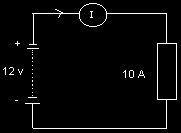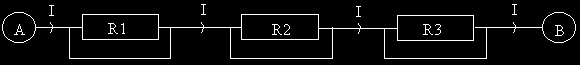Example of Ohm's Law in action……. A ten Ohm resistor is connected across a 12 volt car battery. What current will flow through the resistor? Assume that the battery has no internal resistance. From Ohm's Law: I = V/R = 12/10 = 1.2 Amps. Power is measured in WATTS. It is necessary to be able to calculate the power that is dissipated in various components in an electrical circuit. When too much current passes through a resistor the power is given off in the form of heat. In other words the resistor gets hot'. Some resistors are designed to get hot but normal resistors would be damaged. Power is calculated by multiplying the voltage across the resistor by the current flowing through it. In the above circuit the power dissipated in the resistor would be calculated as follows: There are two other formula for power: W = VV/R or W = I2 R Resistors, as well being made in various values of resistance, are also made in various power ratings. Each value of resistance is usually available in the following wattage ratings: 1/8 W; 1/4 W; 1/2 W; I W; 2 W; 5 W; 10 W; 20 Watts etc. Thus, to be on the safe side, a 20 Watt resistor would be used in the above example. Resistors in seriesIf resistors are connected as above, they are said to be in series. If a voltage is connected across A & B then a current will flow. The current through each resistor will be the same but it must be calculated using the total resistance of the circuit. If the total resistance is known and the total voltage is known then the series current can be easily calculated using Ohm's Law. Let us assume that R1 is 10 Ohms, R2 is 20 Ohms and R3 is 30 Ohms. The total resistance is 10 + 20 + 30 = 60 Ohms. If the supply, connected across A & B, is 12 Volts then the series current will be: I = V/R = 12/60 = 0.2 Amps (or 200 mA) Unless the resistors are all the same value the voltage across each will be different. Knowing the series current and the value of each resistor enables the voltage across resistor to be calculated. Voltage across R1 = I x R1 = 0.2 x 10 = 2 Volts Voltage across R2 = l x R2 = 0.2 x 20 = 4 Volts Voltage across R3 = I x R3 = 0.2 x 30 = 6 Volts You will notice that the sum of these Voltages is equal to the supply Voltage. Thus in a series circuit the voltages are different and the current is the same.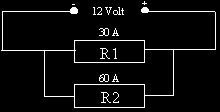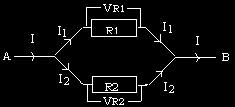The two resistors , in the circuit above, are connected in parallel. In this case the voltage across the resistors will be the same but the current through them will differ. In a parallel circuit the current splits. The lower resistance path will carry the greater current. If the two resistors are the same value the current in each will be half of the total supply current. The formula to find the total resistance of resistors in parallel is :For parallel circuits the total resistance will always be less than any of the individual resistors. Parallel Circuit Example: A 30 Ohm resistor and a 60 Ohm resistor are connected in parallel across a 12 V power supply. What current will flow from the supply assuming it has no internal resistance? First it is necessary to find total resistance Ohm's Law can now be used to find the current. Note that the resultant resistance (20 Ohm) was less than either of the resistors.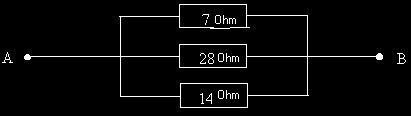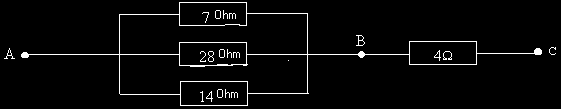QUESTION : 1 (a) What is the difference between a conductor and an insulator? QUESTION : 2 State Ohm's Law QUESTION : 3 (a) A 5 Ohm resistor, a 10 Ohm resistor and a 35 Ohm resistor are connected in series across a home made 100 Volt power supply unit. What current will flow ? (b) How much heat (in Watts) will be dissipated by the 10 Ohm resistor? QUESTION : 4 The regulations state that a "log" must be kept. What details MUST be written down for each QUESTION : 5 Three resistors are connected in parallel as shown. What is the resultant resistance between terminal "A" and terminal "B" ? QUESTION : 6 (a) A 4 Ohm resistor is then added to the above circuit. What is the total resistance between terminal "A" and terminal "C" ? (b) What power will be dissipated by the whole circuit if 50 Volts is connected across "A" and “C” . QUESTION : 7 What, according to the licence, is the purpose of Amateur Radio? QUESTION: 8 What is 0.03 Amps in milliamps (mA) ? QUESTION : 9 What is 0.00002 Volts In microvolts (uV) ? QUESTION : 10 A torch bulb requires 100 mA to light it brightly. How much electricity (in Coulombs) flows if it is turned on for one and a half minutes ?

Source: http://www.sarts.org.sg/rae/Les1.pdf

### Mrten schultz

Mårten Schultz Professor i civilrätt Råsundavägen 156 169 36 SOLNA Utlåtande med anledning av stämningsansökan 1. Börje Ramsbro (i fortsättningen Ramsbro) har hos Stockholms tingsrätt (mål T 7855-12) ansökt om stämning på Stiftelsen Industrifonden (i fortsättningen Industrifonden) med yrkande om att Industrifonden till honom ska erlägga 11,5 miljoner kr för av Industrifonden a

### Bulletin no

BULLETIN NO. 022 Issued May 2000 Revised June 2009 THE RETAIL SALES TAX ACT SALES TO BEEKEEPERS This bulletin outlines the Retail Sales Tax (RST) exemptions on farm implements, machinery and other items used “principally for farming” that are purchased by persons engaged in beekeeping. General • The Retail Sales Tax Act provides an exemption for farm implem